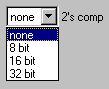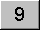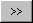calculator.org

# Base-N, Hex, Octal and Binary modes.For calculations involving binary, octal or hexadecimal arithmetic, special modes are used. The calculator is shown in hexadecimal mode. These modes support integer arithmetic only. You can quickly change between number bases using the last row of buttons on the function keypad. Note that earlier versions used four rows of function keys and this will be picked up as the default, so you may need to explicitly set the number of rows to five.

By default, signed numbers are displayed in exactly the same way as in decimal mode, with a minus sign. In computing and logic design it is more common to work with two's complement numbers. To enable two's complement number display use the Options/Display dialog.The two's complement drop-down list allows you to select the word length. Once this is selected, values will be truncated to the selected number of bits for display, with leading zeroes added.The two's complement setting only affects the displayed value. For example, if you keep adding positive values until the value "rolls over", the actual accumulated positive number will be stored internally, although the display will show the truncated value. However, if you switch to decimal mode, or disable two's complement, you will see a large positive number, rather than a smaller negative one. For example, if an 8-bit word length is selected and the decimal number -2 is entered, the value FE will be shown when you convert to hexadecimal. If a decimal of 254 is entered and converted to hexadecimal this will also show FE, but internally this is a different number. The two's complement fixed word length gives you an 8-bit "window" onto the underlying value.

In octal and binary mode some of the buttons are disabled. The button layout for binary mode is shown below, with two's complement and 16-bit word length:For base-n numbers you need to set the number base in Options/Mode. This can be any number base between 2 and 36, and the digits are represented in the range 0-Z. Once the number base is set, it will be remembered whenever Base-n is selected. Setting the number base to ten is useful if you need to use some of the number base or bit wise functions while working with decimal numbers.

For number bases up to 16 (hexadecimal) the 0-9 and A-F buttons gradually become available as required. Beyond this you need to use some kind of keyboard entry to input numbers. The keyboard shortcuts for digits are the capital letters.

Example:

Using Options/Mode, select mode Base-n and set the number base to 22 (you could also set it to a higher number).
Clear the calculator and use the buttons to enter:

 CA

Now use the keyboard to enter L (remembering to use the keyboard shift key).

 CAL

Using the buttons again, enter:

 CALC95

Now do some arithmetic in the normal way, e.g.:

 CALC98

### Logical operators

Logical AND, OR, XOR and XNOR operators are available. They take two operands. There are also unary operators to negate and a NOT operator.

### Bit wise shift operators

Pressing the shift button converts the left shift buttoninto right shift. The shift operator takes two operands - the number to be shifted and the number of bits to be shifted by. It is sometimes inconvenient to specify the number of shift bits in the same number system as the number to be shifted, and you can change number mode during the course of the calculation without affecting the result.

Right shifting a negative number is equivalent to left shifting and vice versa.